﻿ “一带一路”中巴电力合作经济性研究 Economic Research on the BRI Electricity Cooperation with Pakistan

World Economic Research
Vol.06 No.04(2017), Article ID:23005,11 pages
10.12677/WER.2017.64011

Economic Research on the BRI Electricity Cooperation with Pakistan

Changhong Zhao1, Haonan Zhang1, Yu Guo1, Mengya Wu1, Jiahai Yuan1,2*

1School of Economics and Management, North China Electric Power University, NCEPU, Beijing

2Beijing Key Laboratory of New Energy and Low-Carbon Development, North China Electric Power University, Beijing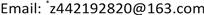Received: Nov. 25th, 2017; accepted: Dec. 5th, 2017; published: Dec. 13th, 2017ABSTRACT

This study uses LCOE model to calculate the cost of electricity coal, hydropower, wind power and photovoltaic project in power cooperation between China and Pakistan. Compared with the local FIT, it is found that electric cooperation projects have the significant economy except the wind power project losing the economy advantage after the FIT of wind power reduction. On this foundation, this study uses project financial evaluation methods to estimate the internal rate of return (IRR) and payback period coal, hydropower and wind power. Finally, the investment environment of electricity market in Pakistan and Indonesia is quantitatively evaluated and contrasted. The critical factors for the electric cooperation between China and Pakistan contain the policy support, security protocol, high FIT and tax incentives, which provides good experience for Chinese power enterprises to go global.

Keywords:Electricity Cooperation, Economy, LCOE, Electric Evaluation

“一带一路”中巴电力合作经济性研究

1华北电力大学，经济与管理学院，北京

2华北电力大学，新能源与低碳发展重点实验室，北京1. 引言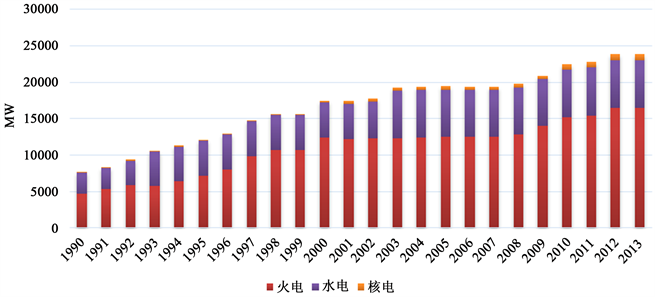Figure 1. Total power installed capacity in Pakistan in 1990-2013

2. 中巴电力合作保障措施与政策

3 电力合作项目经济性

$P=F{\left(1+r\right)}^{-n}$ (1)

$\sum _{n=0}^{N}\frac{Revenue{s}_{n}}{{\left(1+r\right)}^{n}}=\sum _{n=0}^{N}\frac{Cos{t}_{n}}{{\left(1+r\right)}^{n}}$ (2)

$NPV=\sum _{n=0}^{N}PV=0$ (3)

$\sum _{n=0}^{N}\frac{\left(LCO{E}_{n}\right)×\left({E}_{n}\right)}{{\left(1+r\right)}^{n}}=\sum _{n=0}^{N}\frac{Cos{t}_{n}}{{\left(1+r\right)}^{n}}$ (4)

$LCOE=\left(\sum _{n=0}^{N}\frac{Cos{t}_{n}}{{\left(1+r\right)}^{n}}\right)/\left(\sum _{n=0}^{N}\frac{{E}_{n}}{{\left(1+r\right)}^{n}}\right)$ (5)

$LCOE=\left(\sum _{n=1}^{N}\frac{\left(CAPE{X}_{n}+OPE{X}_{n}+TA{X}_{n}\right)}{{\left(1+r\right)}^{n}}\right)/\left(\sum _{n=1}^{N}\frac{{\left(C×H×\left(1-{O}_{u}\right)\right)}_{n}}{{\left(1+r\right)}^{n}}\right)$ (6)

$CAPE{X}_{n}$ ——初始投资成本的年值，包括自有资金、贷款以及折旧；

$OPE{X}_{n}$ ——运维成本的年值，包括燃料、运行维护费用、保险费用、人工成本等；

$TA{X}_{n}$ ——电厂每年应纳税额，包括增值税、所得税、教育附加费、城市维护建设税、土地使用税等；

$C$ ——装机容量， $H$ ——年利用小时数， ${O}_{u}$ ——厂用电率， $N$ ——电厂运营年限， $r$ ——贴现率。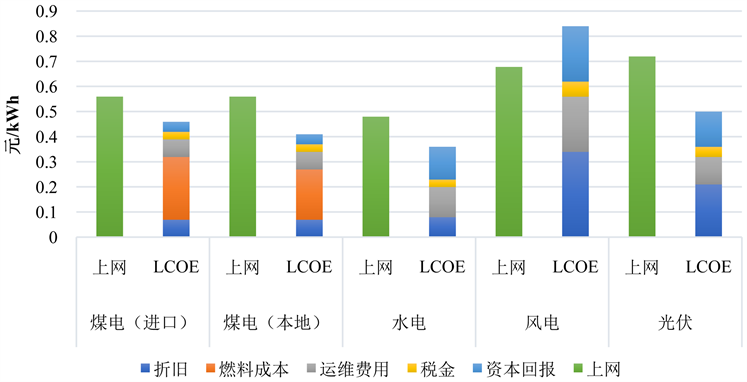Figure 2. Comparison of LCOE and FIT of different generation unitsTable 2. Common parameter setting of LCOE model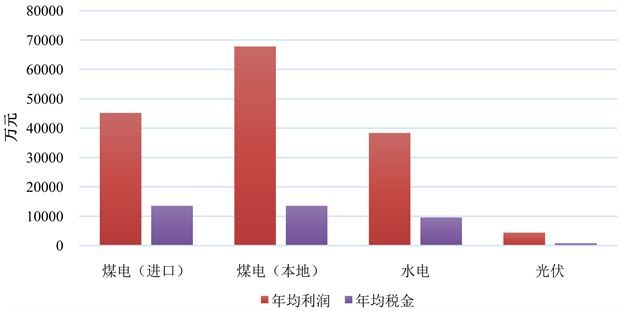Figure 3. Annual profit and tax of electric power projectTable 3. Parameters of hydropower, wind power and photovoltaic

4. 技术经济评价Figure 4. Financial evaluation report compilation

1) 内部收益率(IRR)

2) 投资回收期

5. 电力市场投资环境评价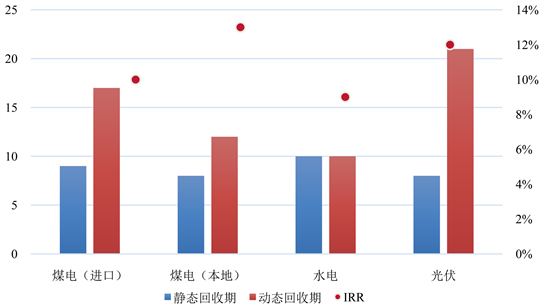Figure 5. Total investment IRR and payback period of different power generation projectsTable 4. Evaluation results of electric power investment environment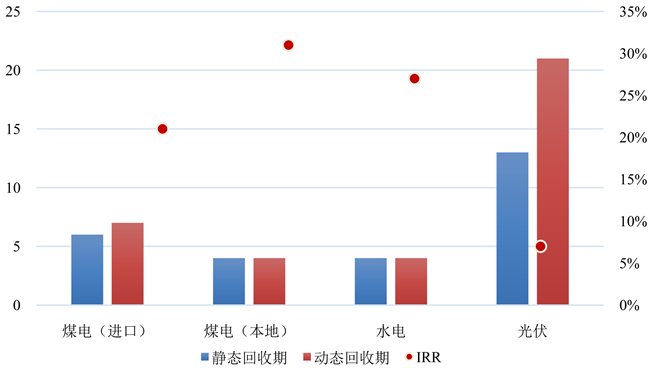Figure 6. IRR of own capital and payback period of investment in different power generation projects

6. 结论与政策建议

Economic Research on the BRI Electricity Cooperation with Pakistan[J]. 世界经济探索, 2017, 06(04): 93-103. http://dx.doi.org/10.12677/WER.2017.64011

1. 1. BP 2016年世界能源统计回顾[R]. 巴黎: BP, 2016.

2. 2. 苏晨. 电力设备“一带一路”: 十年磨一剑, 砺得梅花香[R]. 兴业证券, 2017: 1-66.

4. 4. 和讯网. 巴基斯坦能源危机发酵[EB/OL]. http://news.hexun.com/2015-06-25/177001655.html, 2016.

5. 5. 何时有, 肖欣. “中巴经济走廊”能源电力项目的投资风险[J]. 国际经济合作, 2015(2): 82-85.

6. 6. 祁欣, 王志芳, 等. 以产能合作深化中巴全天候战略伙伴关系[J]. 国际经济合作, 2017(7): 76-81.

7. 7. 中华人民共和国商务部网站. 巴基斯坦电力行业欠费情况继续恶化[EB/OL]. http://www.mofcom.gov.cn/article/i/jyjl/j/201608/20160801374714.shtml

8. 8. 环球网. 巴政府出台政策保障中国电力投资者电费收入[EB/OL]. http://china.huanqiu.com/News/mofcom/2016-02/8582193.html

9. 9. 中研网. 分析: 巴基斯坦面临着严重的电力短缺问题[EB/OL]. http://www.chinairn.com/news/20141022/164356772.shtml

10. 10. 中华人民共和国商务部网站. 巴基斯坦水电发展署欲抛出一系列水电发展优惠政策[EB/OL]. http://www.mofcom.gov.cn/article/i/jyjl/j/201504/20150400942903.shtml

11. 11. 赵长红, 张浩楠, 张兴平, 袁家海. 集中式天然气发电项目经济性研究[J]. 国际石油经济, 2016, 24(12): 57-63.

12. 12. 中华人民共和国驻巴基斯坦伊斯兰共和国大使馆经济商务参赞处. 巴基斯坦大幅下调风电购电价格[EB/OL]. http://pk.mofcom.gov.cn/article/jmxw/201506/20150601024268.shtml

13. 13. 华北电力大学煤电经济性研究课题组. 中国燃煤发电项目的经济性研究[R]. 北京: 华北电力大学, 2016: 1-58.

14. NOTES



*通讯作者。